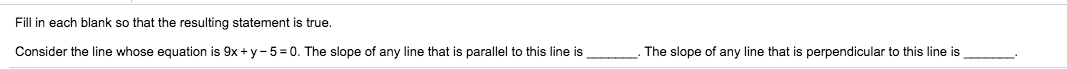# Fill in each blank so that the resulting statement is true.Consider the line whose equation is 9x+ y 50. The slope of any line that is parallel to this line isThe slope of any line that is perpendicular to this line is

Question
9 viewshelp_outlineImage TranscriptioncloseFill in each blank so that the resulting statement is true. Consider the line whose equation is 9x+ y 50. The slope of any line that is parallel to this line is The slope of any line that is perpendicular to this line is fullscreen
check_circle

Step 1

Given, the equation of line is

Step 2

The standard form of line is

Step 3

On comparing equation (1) ...

### Want to see the full answer?

See Solution

#### Want to see this answer and more?

Solutions are written by subject experts who are available 24/7. Questions are typically answered within 1 hour.*

See Solution
*Response times may vary by subject and question.
Tagged in

### Equations and In-equations